## Restraints.spline() — approximate restraints by splines

spline(form, feature, group, spline_dx=0.5, spline_range=4.0, spline_min_points=5, output='', edat=None)
This command calculates and selects new restraints that are a spline approximation of the selected restraints of the specified type. The type is specified by form (see Section 5.3.1), feature (Section 5.3.1) and group (Table 6.1). It unselects the approximated restraints.

The restraint is approximated in a certain range only, determined differently for different mathematical forms:
• Any form acting on an angle feature will be splined from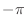to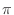.

• forms.gaussian will be splined from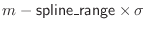to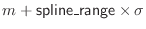, where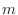is the mean and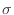the standard deviation.

• forms.multi_gaussian will be splined from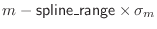to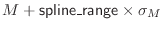, whereand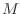are the minimal and maximal means of the basis pdfs, and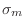and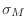are their corresponding standard deviations.

• forms.spline will be splined using the existing range of the spline.

• For user-defined forms (see Section 7.1.2) the range is defined by their get_range function.

• Forms that act on multiple features, such as forms.multi_binormal or suitable user-defined forms, will result in a ValueError.

• All other forms cannot be converted to splines, and will result in a NotImplementedError.

The spline points are distributed evenly over this range with an interval of spline_dx. spline_dx should be equal to the scale of the peaks of the restraint that you want to approximate reliably. The value of the restraint beyond the range is determined by linear extrapolation using the first derivatives at the bounds.

If the x-range and spline_dx are such that the number of spline points would be less than spline_min_points, the high end of the range is increased so that there are spline_min_points defining the “splined” restraint.

If output is set to 'SPLINE', then tables are also written out comparing each pair of original and splined restraints.

Example: See Restraints.make() command.

Automatic builds 2017-07-19